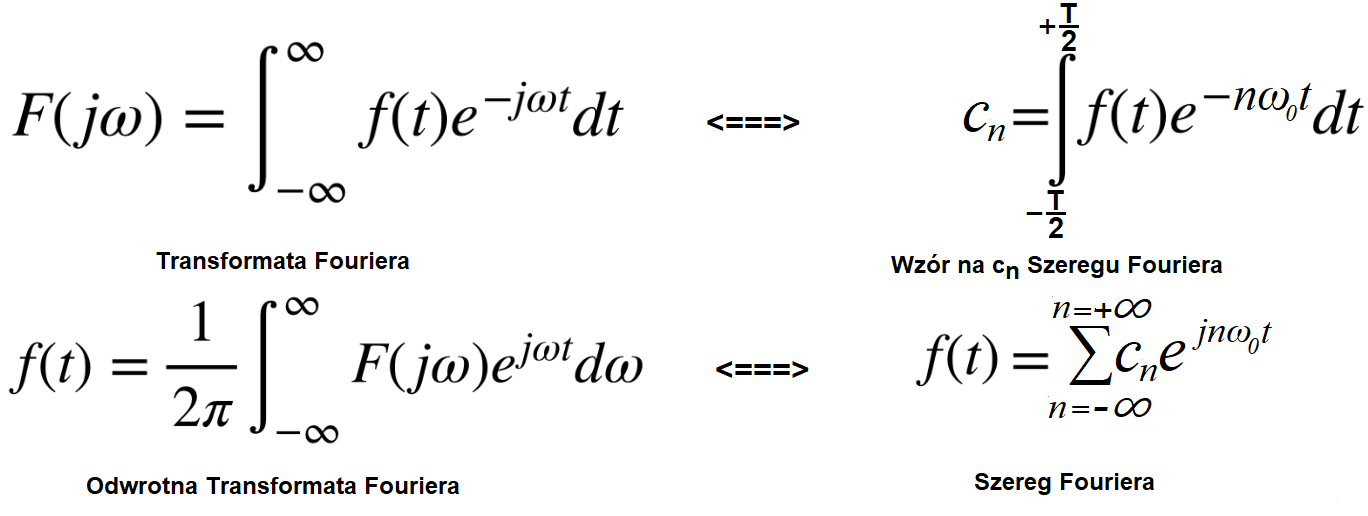### Fourier Transform

Chapter 1 Introduction
We already know that almost every periodic function f(t) can be decomposed into cosines and snusoids with different amplitudes An and Bn and with nω0 pulsations. What about the “normal” functions f(t), meaning non-periodic? It’s similar, only their decomposition into harmonics is more difficult to imagine. Their amplitudes An and Bn are infinitely small. Successive harmonics are infinitely close to each other. Otherwise, their successive pulsations nω0 and (n+1)ω0 are “almost” the same. And the “first harmonic” for ω0=0 is an infinitesimal constant component! For now, the above text may not be entirely clear. I’m sure you will after reading the whole article. But you’ll probably notice the analogy between Series and Fourier Transform.Fig. 1-1
Analogies
1. The F(jω) Fourier Transform is equivalent to the formula for the nth complex amplitude cn of the Fourier Series.
Both, i.e. F(jω) and cn were created on the basis of the time function f(t).

2. The Inverse Fourier Transform is equivalent to the Fourier Series formula. Both rebuild the function f(t) from harmonics.